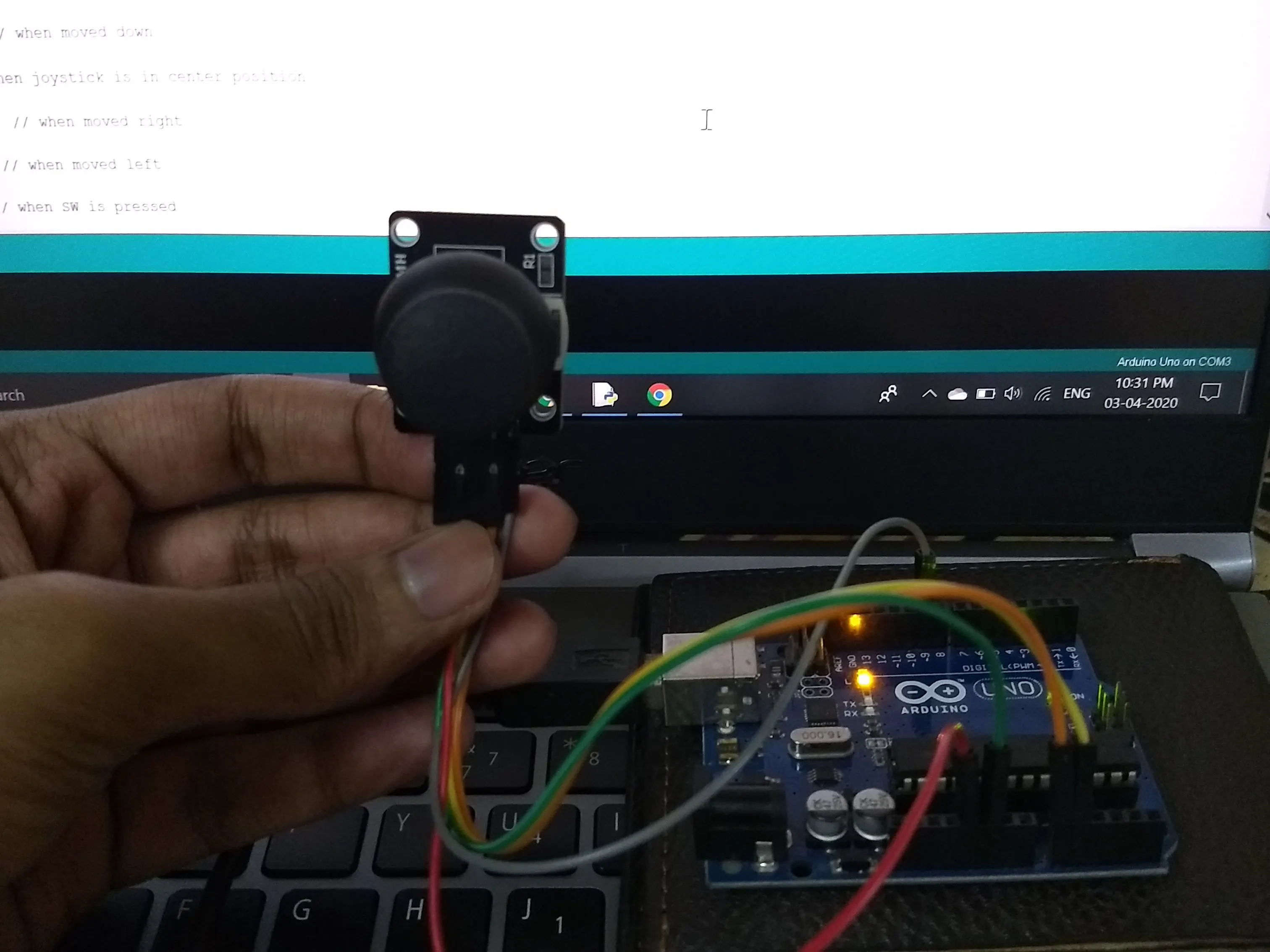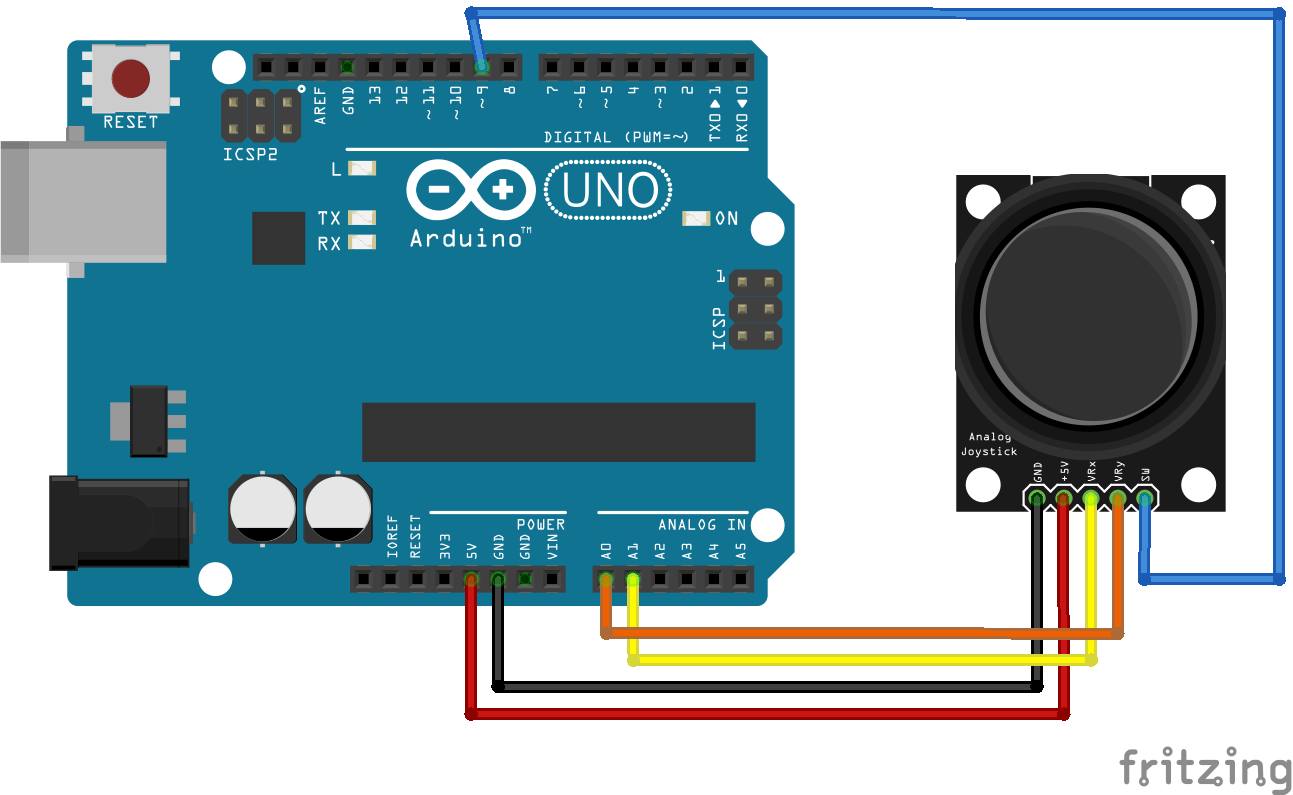Project tutorial# Joystick controlled mouse © GPL3+

Controls cursor using a simple joystick

• 5,438 views
• 41 respects

## Apps and online services

As an alternative to tracking computer's cursor using a mouse or a trackpad, I have made this same application with the help of a joystick. The joystick can move the cursor in any direction (x and Y axis) and also performs click function with the built-in joystick's switch.

### Basics-

A joystick plainly consists of 2 potentiometers aligned in the x and y direction. The arduino reads analog values from the joystick in the range of 0 to 1023. Thus, when the joystick is in its default(center) position, the analog value also becomes close to 500(between 0 and 1023).

The arduino sketch is programmed such that when the joystick is moved away from the center, then print values in the range from -80 to 80 depending on the position. So, when the joystick is moved in one extreme position, the arduino prints value 80 and if the joystick is moved in other extreme position, then -80 is printed.

To print separate values for x and y direction, we will use ":" between the x and y direction values. Example:

The status of joystick button(SW) is printed(1/0) on the serial monitor after the x and y values.

For the laptop/computer to recognise the values, we will need the python's pyautogui module.

### Python Programming

(edited)

The user must have python 3 installed on their laptop/computer. It can be downloaded from the here.

After installation, Copy the path of the python file location.

The following steps are to be performed on the command prompt. Open command prompt and enter the following-

1. `cd <paste the path of the python file>`

2.` py –m pip install –-upgrade pip`

3. `py –m pip install pyautogui`

4. `py -m pip install pyserial`

The pyautogui module is used to perform keyboard/mouse action and pyserial module is used to send/receive data from the arduino. I had already installed the necessary modules, so I got this

The python program is made to read the text printed by the Arduino and recognise the x and y direction values as well as the status of switch(SW).

The current coordinates of the cursor is obtained from the pyautogui function `pyautogui.position()` which provides the cursor's X and Y co-ordinates in the form of pixels.

When the joystick is moved, the analog values(-80 to 80) provided by the arduino is added with the current cursor's position to move the cursor in the desired direction.

To move the cursor in the given direction, the function `pyautogui.moveTo(X+x,Y+y)` satisfies this purpose.

where X and Y is the current cursor's position and x and y are the increment/decrement positions provided by the arduino.

example: `pyautogui.moveTo(100,150)` moves the cursor to 200 pixels in the x-axis and 150 pixels in the y-axis.

To perform click operation based on the SW status, `pyautogui.click(x,y)` is used.

### Final Execution

Upload the arduino sketch(given below) to your arduino UNO and connect the joystick to the arduino pins as given in the schematic.

After ensuring that pyautogui and pyserial is installed on your computer/laptop perform the following steps.

1. Copy the python sketch in a notepad file. Specify the correct COM port of the arduino. From the device manager, you can get the COM port to which the arduino board is connected. Save the file as ".py" after making changes.

2. Open python's IDLE(python GUI) app and open notepad file from it.

2. run the module.

You will then be directed back to screen in Fig 4

In case you see any errors, restart the app and check whether you have mentioned the correct COM port of the arduino.

If there are no errors, then move the joystick and you will see the movement of the cursor.

## Code

##### Arduino CodeArduino
```void setup() {
Serial.begin(9600);
pinMode(9,INPUT);     // SW pin
digitalWrite(9,HIGH);

}

void loop() {
int z=0,xpos=0,ypos=0;
int sensitivity=80;    // you can adjust the sensitivity based on your comfort
if(x>=550)                     // when moved up
xpos=map(x,550,1023,0,sensitivity);
if(x<=450)                   // when moved down
xpos=map(x,450,0,0,-sensitivity);
if(y>=550)                    // when moved right
ypos=map(y,550,1023,0,sensitivity);
if(y<=450)                  // when moved left
ypos=map(y,450,0,0,-sensitivity);
if(digitalRead(9)==LOW)   // when SW is pressed
z=1;
else
z=0;
if(xpos!=0 or ypos!=0 or z==1) // prints only when the joystick is moved or pressed
{
Serial.print(xpos);    // print the data and separating by ":"
Serial.print(":");
Serial.print(ypos);
Serial.print(":");
Serial.println(z);
}
delay(100);         // for normal operation
}
```
##### Python CodePython
```import pyautogui, sys
import time
import serial

pyautogui.FAILSAFE=False
ArduinoSerial=serial.Serial('com3',9600)  #Specify the correct COM port
time.sleep(1)                             #delay of 1 second

while 1:
(x,y,z)=data.split(":")           # assigns to x,y and z
(X,Y)=pyautogui.position()        #read the cursor's current position
x=int(x)                           #convert to int
y=int(y)
pyautogui.moveTo(X+x,Y-y)           #move cursor to desired position
if '1' in z:                        # read the Status of SW
pyautogui.click(button="left")    # clicks left button

```

## Schematics#### Author• 5 projects
• 15 followers

April 4, 2020

#### Members who respect this project

See similar projects
you might like

• 1,597 views
• 1 comment
• 8 respects

#### Joystick Controlled RC Car

Project tutorial by Arduino_Scuola

• 17,383 views
• 18 respects

#### Robot Rover - iPhone controlled using Blynk Joystick

Project tutorial by jim Myracle

• 7,569 views
• 19 respects

#### Laser guidance using Joystick

Project showcase by Shubham Santosh

• 1,728 views
• 9 respects

#### Mobile Controlled Bluetooth Car || Easy || Simple || HC-05

Project tutorial by Team Vishal soni

• 27,580 views